Rational mapping

A generalization of the concept of a rational function on an algebraic variety. Namely, a rational mapping from an irreducible algebraic varietyto an algebraic variety(both defined over a field) is an equivalent class of pairs, whereis a non-empty open subset ofandis a morphism fromto. Two pairs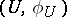and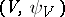are said to be equivalent if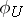and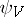coincide on. In particular, a rational mapping from a varietyto an affine line is a rational function on. For every rational mapping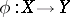there is a pair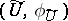such that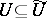for all equivalent pairsandis the restriction ofto. The open subset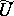is called the domain of regularity of the rational mapping, and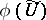is the image of the variety(written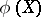) under.
Ifis a rational mapping of algebraic varieties andis dense in, thendetermines an imbedding of fields,. Conversely, an imbedding of the fields of rational functionsdetermines a rational mapping fromto. Ifinduces an isomorphism of the fields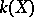and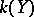of rational functions, thenis called a birational mapping.
The set of points ofat which the rational mappingis not regular has codimension 1, in general. But ifis complete andis smooth and irreducible, then this set has codimension at least 2. Ifandare complete irreducible varieties over an algebraically closed field of characteristic 0, then the rational mappingcan be included in a commutative diagram (see ):(*)
where,are morphisms of an algebraic variety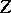andis a composite of monoidal transformations (cf. Monoidal transformation). Ifis a birational mapping of complete non-singular surfaces, then there exists a diagram (*) in which bothandare composites of monoidal transformations with non-singular centres (Zariski's theorem), that is, every birational mapping of complete non-singular surface decomposes into monoidal transformations with non-singular centres and their inverses. In the case when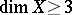, the question of whether every birational mapping can be decomposed in this way is open (1990).